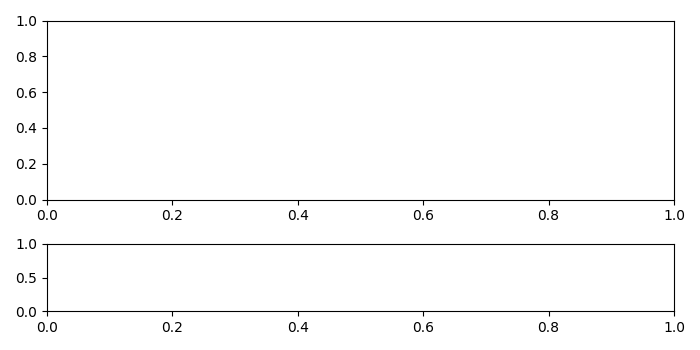# Changing Matplotlib subplot size/position after axes creation

To change subplot size or position after axes creation, we can take the following steps−

• Create a new figure or activate an existing figure using figure() method.
• Add an '~.axes.Axes' to the figure as part of a subplot arrangement using add_subplot() method.
• A grid layout to place subplots within a figure using GridSpec() class.
• Set the position of the grid specs.
• Set the subplotspec instance.
• Add an '~.axes.Axes' to the figure as part of a subplot arrangement using add_subplot() method, with gridspec instance.
• To display the figure, use show() method.

## Example

from matplotlib import pyplot as plt
from matplotlib import gridspec as gridspec
plt.rcParams["figure.figsize"] = [7.00, 3.50]
plt.rcParams["figure.autolayout"] = True
fig = plt.figure()
plt.show()